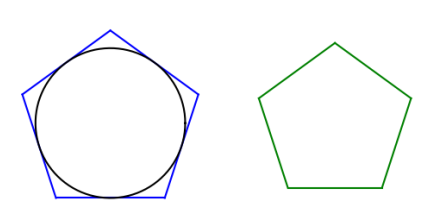# Galileo’s polygon theorem

William J. Milne  attributes the following theorem to Galileo:

The area of a circle is a mean proportional between the areas of any two similar polygons, one of which is circumscribed about the circle and the other is isoparametric with the circle.

So imagine a polygon P and let S be an inscribed circle, that is, the largest circle that can fit inside P. Also imagine a second polygon P‘ the same shape as P but with perimeter equal to that of S. Then the ratio of the area of P to the area of S equals the ratio of the area of S to the area of P‘. Said another way, the area of S is the geometric mean between the areas of P and P‘.In the figures above, the blue pentagon is P, the black circle is S, the green pentagon is P‘, and S and P‘ have the same perimeter.

Clearly the blue pentagon has a greater area than the black circle. The isoparametric theorem says that a circle has the maximum area for a given perimeter, so the black circle must have larger area than the smaller pentagon. This doesn’t prove Galileo’s theorem, but it does prove that at least the area of the circle is somewhere in between the areas of the two pentagons.

You can prove Galileo’s theorem for regular n-gons using the formulas in this post and one other fact: the ratio of the radii of inscribed and circumscribed circles sandwiching a regular n gon is cos(π/n).

 William J. Milne. Plane and Solid Geometry. 1899. p. 377, exercise 135.

## 2 thoughts on “Galileo’s polygon theorem”

1. Nathan Hannon

This certainly can’t be true for all polygons. For example, you could add a very long and narrow protrusion to the polygon P, which would have no effect on the area of S and an arbitrarily small effect on the area of P, but would make the perimeter of P arbitrarily large and hence the area of P’ arbitrarily small. Does this require all the edges of the polygon to be tangent to the circle?

2. Daniel

To respond to Nathan, it does require that all edges of the polygon be tangent to the circle. In the theorem, it already states that the polygon must be circumscribed about the circle, which means exactly that.